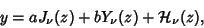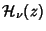## Struve Differential Equation

The ordinary differential equationwhereis the Gamma Function. The solution iswhereandare Bessel Functions of the First and Second Kinds, andis a Struve Function (Abramowitz and Stegun 1972).

See also Bessel Function of the First Kind, Bessel Function of the Second Kind, Struve Function

References

Abramowitz, M. and Stegun, C. A. (Eds.). Handbook of Mathematical Functions with Formulas, Graphs, and Mathematical Tables, 9th printing. New York: Dover, pp. 496, 1972.